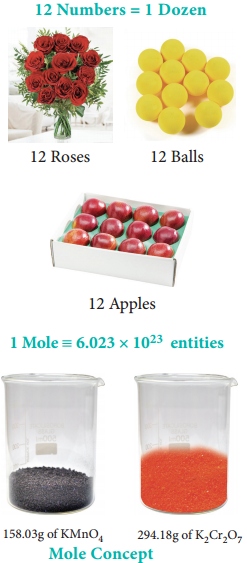Find free online Chemistry Topics covering a broad range of concepts from research institutes around the world.

## Mole Concept – What is a Mole? Related Formulae and Examples

Often we use special names to express the quantity of individual items for our convenience. For example, a dozen roses means 12 roses and one quire paper means 24 single sheets. We can extend this analogy to understand the concept of mole that is used for quantifying atoms and molecules in chemistry. Mole is the SI unit to represent a specific amount of a substance.

To understand the mole concept, let us calculate the total number of atoms present in 12 g of carbon – 12 isotope or molecules in 158.03 g of potassium permanganate, 294.18 g of potassium dichromate and 180 g of glucose.Table 1.2 Calculation of Number of Entities in One Mole of Substance.From the calculations we come to know that 12 g of carbon-12 contains 6.022 × 1023 carbon atoms and same numbers of molecules are present in 158.03 g of potassium permanganate and 294.18 g of potassium dichromate. Similar to the way we use the term ‘dozen’ to represent 12 entities, we can use the term ‘mole’ to represent 6.022 × 1023 (atoms or molecules or ions)

One mole is the amount of substance of a system, which contains as many elementary particles as there are atoms in 12 g of carbon-12 isotope. The elementary particles can be molecules, atoms, ions, electrons or any other specified particles.